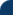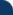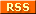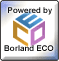Delphi Clinic C++Builder Gate Training & Consultancy Delphi Notes Weblog Dr.Bob's WebshopThese are the voyages using Delphi Enterprise (and Architect). Its mission: to explore strange, new worlds. To design and build new applications. To boldly go...Title: Author: Bob Swart Posted: 12/4/2008 9:24:36 AM (GMT+1) Content: SysUtils defines the well-known IntToStr and FloatToStr, which return a Unicode String - but not an AnsiString. If you want to use AnsiStrings in your application, you may want to use versions of these routines that return an AnsiString. Since these didn’t exist, yet, I’ve written a set of routines to help us converting integers and floats to AnsiStrings, based on the built-in Str function.`unit Convert;interface function IntToAnsiStr(X: Integer; Width: Integer = 0): AnsiString; function FloatToAnsiStr(X: Extended; Width: Integer = 0; Decimals: Integer = 0): AnsiString; overload;function FloatToAnsiStr(X: Double; Width: Integer = 0; Decimals: Integer = 0): AnsiString; overload;function FloatToAnsiStr(X: Single; Width: Integer = 0; Decimals: Integer = 0): AnsiString; overload; implementation function IntToAnsiStr(X: Integer; Width: Integer = 0): AnsiString;begin Str(X: Width, Result);end; function FloatToAnsiStr(X: Extended; Width: Integer = 0; Decimals: Integer = 0): AnsiString;begin Str(X: Width: Decimals, Result);end; function FloatToAnsiStr(X: Double; Width: Integer = 0; Decimals: Integer = 0): AnsiString;begin Str(X: Width: Decimals, Result);end; function FloatToAnsiStr(X: Single; Width: Integer = 0; Decimals: Integer = 0): AnsiString;begin Str(X: Width: Decimals, Result);end; end.`Note that the overloaded FloatToAnsiStr function uses default Width and Decimal arguments (with a default value of 0). Also note that the Str function always uses the dot as decimal separator in the string result, and doesn’t consider the DecimalSeparator value. If you want to use the DecimalSeparator, you may want to change the functions as follows:`function FloatToAnsiStr(X: Double; Width: Integer = 0; Decimals: Integer = 0): AnsiString;const Dot: AnsiChar = '.';begin Str(X: Width: Decimals, Result); if DecimalSeparator <> '.' then begin Decimals := AnsiPos(Dot, Result); if Decimals > 0 then Result[Decimals] := AnsiChar(DecimalSeparator) endend;`This will use the DecimalSeparator, narrowed back to AnsiChar (and I’m assuming nobody will use a Unicode Character which doesn’t “map” back to an AnsiChar as DecimalSeparator, but if you do, you could just stick to the normal FloatToStr anyway).This tip is the 8th in a series of Unicode tips taken from my Delphi 2009 Development Essentials book published earlier this week on Lulu.com. Back